## Study Questions

— With QuizzerWeb

##### MATHEMATICS (2016) QUIZ STUDY
The Correct Options are highlighted thus - >
Correct Answer
.

Please share this quiz link to your friends, they might need it! Click here to Practice MATHEMATICS (2016) in CBT mode

1.
Integrate $$\frac{2x^3 + 2x}{x}$$ with respect to x
.
A. $$\frac{2x^3}{3}$$ - 2x + k
B. x3 + 2x + k
C. $$\frac{2x^3}{3}$$ + 2x + k
D. x3 - 2x + k
Explanation.
$$\int$$ $$\frac{2x^3 + 2x}{x}$$ = $$\int$$ $$\frac{2x^3}{x}$$ + $$\frac{2x}{x}$$ = $$\int$$ (2x2 + 2) dx

$$\frac{2x^2+1}{2 + 1}$$ + 2x + k

= $$\frac{2x^3}{3}$$+ 2x + k

2.
If the mean of 4, y, 8 and 10 is 7. Find Y?
.
A. 6
B. 10
C. 7
D. 9
Explanation.
$$\frac{4 + y + 8 + 10}{4}$$ = 7

22 + y = 4 x 7
22 + y = 28
y = 28 - 22 = 6

3.

Find the derivative of y = ( $$\frac{1}{3}$$X + 6)

.
A.

2( $$\frac{1}{3}$$X + 6 )

B.

$$\frac{2}{3}$$ ( $$\frac{1}{3}$$X + 6)

C.

$$\frac{2}{3}$$ ( $$\frac{1}{3}$$X + 6)2

D.

$$\frac{1}{3}$$ ( $$\frac{1}{3}$$X + 6)2

Explanation.

Y = ( $$\frac{1}{3}$$X + 6)2

$$\frac{dy}{dx}$$ = 2( $$\frac{1}{3}$$X + 6) $$\frac{1}{3}$$

= $$\frac{2}{3}$$ ( $$\frac{1}{3}$$X + 6)

4.
In a school of 150 students, 80 offer French while 60 offer Arabic and 20 offer neither. How many students offer both subjects?
.
A. 45
B. 10
C. 35
D. 30
Explanation.
80 - x + x + 60 - x + 20 = 150
160 - x = 150
x = 160 - 150 = 10

5.
If the 2nd term of a G.P is $$\frac{8}{9}$$ and the 6th term is 4$$\frac{1}{2}$$. Find the common ratio.
.
A. 2
B. $$\frac{3}{2}$$
C. $$\frac{2}{3}$$
D. 3
Explanation.
T2 = $$\frac{8}{9}$$, T6 = 4$$\frac{1}{2}$$
ar = $$\frac{8}{9}$$
ar5 = $$\frac{9}{2}$$

$$\frac{ar}{ar^5}$$ = $$\frac{8}{9}$$ x $$\frac{2}{9}$$

$$\frac{1}{r^4}$$ = $$\frac{16}{81}$$

r = $$\sqrt{18/16}$$ = $$\frac{3}{2}$$

6.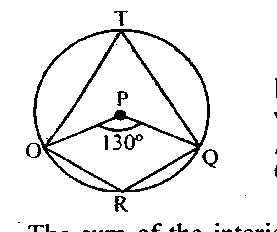From the diagram above, find the value of < OTQ

.
A.

230o

B.

55o

C.

115o

D.

65o

Explanation.
< OTQ = $$\frac{130}{2}$$
= 65o

7.
The sum of the interior angles of a polygon is a given as 1080o. Find the number of the sides of the polygon.
.
A. 5
B. 7
C. 6
D. 8
Explanation.
$$\frac{(n - 2) 180}{180}$$ = $$\frac{1080}{180}$$

(n - 2) = 6
n = 6 + 2 = 8

8.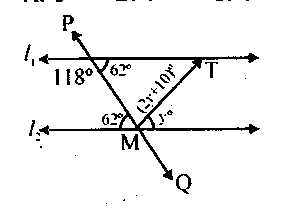In the diagram above, l1 is parallel to l2, Find the value of < PMT

.
A.

82o

B.

36o

C.

72o

D.

118o

Explanation.
< MPT = 180o - 118o = 62o

< PML = 62o ( Alternative angle)

y + 2y + 10o + 62o = 180o (Angles on a straight line)

3y = 180 - 72
$$\frac{3y}{3}$$ = $$\frac{108}{3}$$
y = 36o

< PMT = 2y + 10 = 2(36) + 10 = 82o

9.From the diagram above, Find the value of < ROP

.
A.

110o

B.

70o

C.

95o

D.

85o

Explanation.
< ROP = 95o (Exterior angle of cyclic quadrilateral)

10.The venn diagram shows a class of 50 students with the games they play. How many students play only two games?

.
A.

15

B.

16

C.

20

D.

18

Explanation.

Number of students that plays only two games = 4 + 8 + 3  = 15

11.
If line p = 5x + 3 is parallel to line p = wx + 5. Find the value of w.
.
A. 7
B. 3
C. 6
D. 5
Explanation.
P = 5x + 3, P = wx + 5
M1 = 5, M2 = w
M1 = M2 (parallel lines)
w = 5

12.
Evaluate $$\int$$(cos4x + sin3x)dx
.
A. sin4x - cos3x + k
B. sin4x + cos3x + k
C. $$\frac{1}{4}$$sin4x - $$\frac{1}{3}$$cos3x + k
D. $$\frac{1}{4}$$sin4x + $$\frac{1}{3}$$cos3x + k

13.
If x10 = 235. Find x
.
A. 15
B. 12
C. 14
D. 13
Explanation.
x10 = 235
x10 = 2 x 51 + 3 x 50
= 10 + 3
= x10 = 13

14.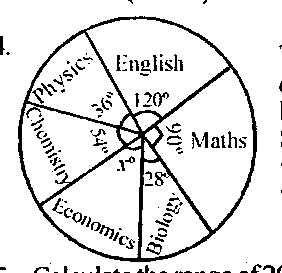The pie chart above shows the distribution of subjects offered by students in SSS III level. If 80 students enrolled in the class. What is the size of the angle of the sector in economics?

.
A.

24o

B.

39o

C.

32o

D.

36o

Explanation.
xo + 36o + 54o + 28o + 90o + 120o = 360o

x + 328o = 360o
x = 360o - 328o
x = 32o

15.
Calculate the range of 20, -6, 25, 30, 21, 28, 32, 33, 34, 5, 3, 2, and 1.
.
A. 32
B. 36
C. 33
D. 40
Explanation.
Range = 32- (-6) = 34 + 6 = 40

16.The bar chart above shows the number of visitors received in a week. How many visitors were received on Friday, Tuesday and Sunday?
.
A. 17
B. 22
C. 20
D. 16
Explanation.
No of visitors = 10 + 5 + 1 = 16

17.
Factorize k2 - 2kp + p2.
.
A. (k +p)2
B. (k - p)2
C. k2 + p2
D. k2 - p2
Explanation.
k2 - 2kp + p2 = (k - p)2

18.
Calculate the perimeter of a sector of a circle of raduis 12cm and angle 60o.
.
A. (12 + 4$$\pi$$)cm
B. (24 + 4$$\pi$$)cm
C. (12 + 6$$\pi$$)cm
D. (24 + 6$$\pi$$)cm
Explanation.
Perimeter = 2r + $$\frac{0}{360}$$ x 2$$\pi$$r
= 2(12) + $$\frac{60}{360}$$ x 2 x $$\pi$$ x 12
= (24 + 4$$\pi$$)cm

19.
$$\begin{array}{c|c} Marks & 2 & 3 & 4 \\ \hline Frequency & 4 & 4 & y \end{array}$$

The table above shows the frequency distribution of marks obtained by a group of students. If the total mark is 48, find the value of y.
.
A. 6
B. 8
C. 7
D. 5
Explanation.
(2 x 4) + (3 x 4) + (4 x y) = 48
8 + 12 + 4y = 48
4y = 48 - 20
$$\frac{4y}{4}$$ = $$\frac{28}{4}$$
y = 7

20.
Given U = {xx is a positive integer less than 15} and P = {xx is even number from 1 to 14}. Find the compliment
.
A. {1, 3, 5, 7, 9, 11, 13, 15}
B. {2, 3, 5, 7, 9, 11, 13}
C. {1, 3, 5, 7, 9, 11, 13}
D. {2, 3, 5, 7, 11, 15}
Explanation.
u = {1, 2, 3, 4, 5......14}
p = {2, 4, 6, 8, 10, 12, 14}
p1 = {1, 3, 5, 7, 9, 11,13}

21.
Simplify $$\frac{0.026 \times 0.36}{0.69}$$. Leave your answer in standard form
.
A. 1.36 x 10-4
B. 1.36 x 10-3
C. 1.36 x 10-1
D. 1.36 x 10-2
Explanation.
$$\frac{0.026 \times 0.36}{0.69}$$ = $$\frac{26 \times 10^-3 \times 36 \times 10^-2}{69 \times 10^-2}$$

= 0.01356 = 1.36 x 10-2

22.
A number of pencils were shared out among Bisi, Sola and Tunde in the ratio of 2:3:5 respectively. If Bisi got 5, how many were shared out?
.
A. 15
B. 25
C. 30
D. 50
Explanation.
Let x represent total number of pencils shared
B : S : T
2 : 3 : 5

2+3+5 = 10
$$\frac{2}{10}$$ x x = 5
$$\frac{2x}{10}$$ = 5
2x = 50
x = 25

23.
Calculate the perimeter of a sector of a circle of raduis 9cm and angle 36o.
.
A. 18cm
B. (18 + $$\frac{9\pi}{5}$$)cm
C. (9 + $$\frac{9\pi}{5}$$)cm
D. $$\frac{9\pi}{5}$$cm
Explanation.
Perimeter = 2r + $$\frac{\theta}{360}$$ x 2$$\pi$$r
2(9) + $$\frac{36}{360}$$ x 2 x $$\pi$$ x 9 = 18 + $$\frac{9\pi}{5}$$

24.
Evaluate $$\frac{27^\frac{1}{3} - 8^\frac{2}{3}}{16^\frac{2}{4} \times 2}$$
.
A. $$\frac{23}{5}$$
B. -$$\frac{23}{5}$$
C. $$\frac{1}{8}$$
D. $$\frac{21}{7}$$
Explanation.
$$\frac{27^\frac{1}{3} - 8^\frac{2}{3}}{16^\frac{2}{4} \times 2}$$

$$\frac{27^\frac{1}{3} - 8^\frac{2}{3}}{16^\frac{2}{4} \times 2}$$ = $$\frac{3\sqrt{27} - 3\sqrt{8}^2}{\sqrt{16} \times 2}$$

$$\frac{3 - 4}{4 \times 2}$$
= $$\frac{-1}{8}$$

25.
$$\begin{array}{c|c} Scores & 3 & 6 & 5 & 2 \\ \hline Frequency & 2 & 3 & 4 & 6 \end{array}$$

From the table above, find the median
.
A. 3
B. 5
C. 4
D. 6
Explanation.
Mean = $$\frac{(2 \times 3) + (3 \times 6) + (4 \times 5) + (2 \times 6)}{2 + 3 + 4 + 6}$$

= $$\frac{6 + 18 + 20 + 12}{15}$$ = $$\frac{56}{15}$$

= 3.73

26.
Find $$\frac{dy}{dx}$$, if y = $$\frac{2}{3}$$ X3 - $$\frac{4}{x}$$
.
A. 3x2 - $$\frac{4}{x}$$
B. 2x2 +$$\frac{4}{x^2}$$
C. 3x2 + $$\frac{4}{x^2}$$
D. 2x2 - $$\frac{4}{x}$$
Explanation.
y = $$\frac{2}{3}$$x3 - $$\frac{4}{x}$$

$$\frac{dy}{dx}$$ = 2x2 - Lx-2 = 2x2 + $$\frac{4}{x^2}$$

27.
Evaluate $$\frac{0.8 \times 0.43 \times 0.031}{0.05 \times 0.72 \times 0.021}$$. Correct to four significant figures.
.
A. 14.09
B. 14.1
C. 14.12
D. 14.11
Explanation.
$$\frac{0.8 \times 0.43 \times 0.031}{0.05 \times 0.72 \times 0.021}$$ x $$\frac{10}{10}$$ = 14.11

28.
An arc subtends an angle of 30o at the centre of a circle radius 12cm. Calculate the length of the arc.
.
A. 6$$\pi$$cm
B. 2$$\pi$$cm
C. 3$$\pi$$cm
D. 9$$\pi$$cm
Explanation.
Length or arc = $$\frac{\theta}{360}$$ x 2$$\pi$$r

= $$\frac{30}{360}$$ x 2 x $$\frac{22}{7}$$ x 12

= $$\frac{1}{12}$$ x 2 x $$\pi$$ x 2 = 2$$\pi$$cm

29.
Evaluate $$\int\limits_0^\frac{\pi}{2}$$ sin xdx
.
A. 2
B. -1
C. 1
D. -2
Explanation.
$$\int\limits_0^\frac{\pi}{2}$$ sin xdx = -cosx|$$\frac{\pi}{2}$$

= -(cos$$\frac{\pi}{2}$$ - cos0) = -(0-1) = 1

30.
An arc of the length 16$$\pi$$cm subtends an angle of 80o at the centre of the circle. Find the radius of the circle.
.
A. 24cm
B. 28cm
C. 36cm
D. 32cm
Explanation.
L = $$\frac{\theta}{360}$$ x 2$$\pi$$r
16$$\pi$$ = $$\frac{80}{360}$$ x 2$$\pi$$r

$$\frac{16\pi \times 360}{80 \times 2\pi}$$ = r

r = 36cm

31.

The mean of 2-t, 4+t, 3-2t, 2+t and t-1 is

.
A.

2

B.

t

C.

-t

D.

-2

Explanation.

Mean = $$\frac{2 - t + 4 + t + 3 - 2t + 2 + t + t - 1}{5}$$

= $$\frac{10}{5}$$ = 2

32.
Evaluate $$\frac{12.02 \times 20.06}{26.04 \times 60.06}$$, correct to three significant figures.
.
A. 0.157
B. 0.154
C. 0.155
D. 0.158
Explanation.
$$\frac{12.02 \times 20.06}{26.04 \times 60.06}$$ = $$\frac{241.1212}{15698.96}$$ = 0.154

33.
If y = 2x3 + 6x2 + 6x + 1, Find $$\frac{dy}{dx}$$
.
A. 6x2 + 12x + 1
B. 6x2 + 6x + 1
C. 6x2 + 6x + 6
D. 6x2 + 12x + 6
Explanation.
y = 2x3 + 6x2 + 6x + 1

$$\frac{dy}{dx}$$ = 6x2 + 12x + 6

34.
The nth term of the sequence 3, 9, 27, 81.....is
.
A. 3 x 3n-2
B. 3 x 3n-1
C. 3 x 3n+2
D. 3 x 3n+1
Explanation.
3,9,27,81,.....
r = $$\frac{9}{8}$$ = 3
Tn = arn-1

Tn = 3(3)n-1 = $$\frac{3(3^2)}{3}$$

35.
Simplify 1 - ($$\frac{1}{7}$$ x 3 $$\frac{1}{2}$$) $$\div$$ $$\frac{3}{4}$$
.
A. 2
B. $$\frac{1}{3}$$
C. 1
D. $$\frac{2}{3}$$
Explanation.
1 - ($$\frac{1}{7}$$ x 3 $$\frac{1}{2}$$) $$\div$$ $$\frac{3}{4}$$

1 - ($$\frac{1}{7}$$ x $$\frac{7}{2}$$) x $$\frac{4}{3}$$

1 - $$\frac{1}{2}$$ x $$\frac{4}{3}$$

1 - $$\frac{2}{3}$$ = $$\frac{1}{3}$$

36.
If a car travels 120km on 45 litres of petrol, how much petrol is needed for a journey of 600km?
.
A. 720 litres
B. 160 litres
C. 225 litres
D. 960 litres
Explanation.
120km $$\to$$ 45litres
1km $$\to$$ $$\frac{45}{120}$$litres
600km $$\to$$ $$\frac{45}{120}$$ x 600 = 225litres

37.
Find $$\frac{dy}{dx}$$. If y = 3x3 + 2x2 + 3x + 1
.
A. 9x2 + 4x + 3
B. 9x2 - 4x - 3
C. 9x2 + 4x - 3
D. 9x2 - 4x + 3
Explanation.
y = 3x3 + 2x2 + 3x + 1
$$\frac{dy}{dx}$$ = 9x2 + 4x + 3

38.
Evaluate $$\int$$(sinx - 5x2)dx
.
A. -cosx - 10x + k
B. cosx - $$\frac{5x^3}{3}$$ + k
C. -cosx - $$\frac{5x^3}{3}$$ + k
D. cosx - 10x + k
Explanation.
$$\int$$(sin x - 5x^2) = -cosx - $$\frac{5x^3}{3}$$ + k

39.

If N = $$\frac{p}{2}$$($$\frac{T_1 - T_2}{T_1}$$). Find P when N = 12, T1 = 27 and T2 = 24.

.
A.

48

B.

108

C.

54

D.

216

Explanation.

N = $$\frac{p}{2}$$($$\frac{T_1 - T_2}{T_1}$$)
12 = $$\frac{p}{2}$$($$\frac{27 - 24}{27}$$)
24 = P($$\frac{3}{27}$$)
P = 24 x 9 = 216

40.
Solve for x and y respectively
3x - 5y = 9
6x - 4y = 12
.
A. $$\frac{3}{4}$$, 1
B. $$\frac{4}{3}$$, 1
C. $$\frac{3}{4}$$, -1
D. $$\frac{4}{3}$$, -1
Explanation.
3x - 5y = 9 ------x2
6x - 4y = 12 -----x1
6x - 10y = 18
-6x - 4y = 12
____________
$$\frac{6y}{-6}$$ = $$\frac{6}{6}$$

y = -1
in eq (1) 3x - 5y = 9
3x - 5(-1) = 9
3x + 5 = 9
3x = 4
x = $$\frac{4}{3}$$

41.
If Q is a factor of 18 and T is prime numbers between 2 and 18. What is Q$$\cap$$T?
.
A. (2,3)
B. (2,3,18)
C. (2,3,9)
D. (2,3,6)
Explanation.
Q = {1,2,3,6,9,18}, T = {2,3,5,7,11,13,17}
Q$$\cap$$T = {2,3}

42.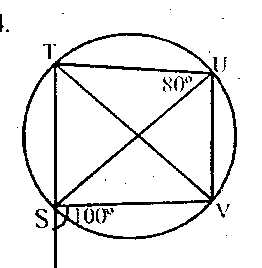From the cyclic quadrilateral above, find < TVS

.
A.

60o

B.

80o

C.

70o

D.

50o

Explanation.
< TVS = 180o - (80 + 20)
= 180 - 100 = 80o

43.
Find the mean of 10, 8, 5, 11, 12, 9, 6, 3, 15, and 23.
.
A. 11.2
B. 10
C. 10.2
D. 11.3
Explanation.
$$\frac{10 + 8 + 5 + 11 + 12 + 9 + 6 + 3 + 15 + 23}{10}$$
= $$\frac{102}{10}$$ = 10.2

44.In the cyclic quadrilateral above . Find < PRO

.
A.

70o

B.

20o

C.

50o

D.

30o

Explanation.
< PRO = 180o - (100 + 50)
= 180 - 150 = 30o

45.
OGIVE is constructed using
.
A. Third quartile range
B. Semi-quartile range
C. Cummulative frequency table
D. Inter-quartile table

46.
Rationalize $$\frac{\sqrt{6} - \sqrt{4}}{\sqrt{6} + \sqrt{4}}$$
.
A. 5 + 2 $$\sqrt{6}$$
B. 5 - 4 $$\sqrt{6}$$
C. 5 + 4 $$\sqrt{6}$$
D. 5 - 2 $$\sqrt{6}$$
Explanation.
$$\frac{\sqrt{6} - \sqrt{4}}{\sqrt{6} + \sqrt{4}}$$ = $$\frac{6-2\sqrt{6} - 2\sqrt{6} + 4}{6 - 4}$$

$$\frac{10 - 4\sqrt{6}}{2}$$ = 5 - 2$$\sqrt{6}$$

47.The bar chart above shows the marks obtained by students in a mathematics test.
How many students in all took the test?

.
A.

40

B.

30

C.

10

D.

20

Explanation.
3 + 2 + 5 + 5 + + 5 + 10 + 4 + 3 + 1 + 2 = 40

48.Determine the mean score of the student that took the mathematics test?

.
A.

4.5

B.

4.3

C.

4.2

D.

4.6

Explanation.
(3 x 0) + (1 x 2) + (5 x 2) + (5 x 3) + (5 x 4) +
(10 x 5) + (4 x 6) + (3 x 7) + (1 x 8) + (2 x 9) $$\div$$ 40

= $$\frac{168}{40}$$ = 4.2

49.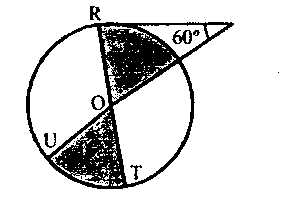From the diagram above. Find the fraction of the shaded position?

.
A.

$$\frac{1}{3}$$

B.

$$\frac{1}{5}$$

C.

$$\frac{1}{4}$$

D.

$$\frac{1}{6}$$

Explanation.
$$\theta$$ = 180o -(90 + 60)
$$\theta$$ = 180o - 150o = 30o

Fraction of shaded position = $$\frac{30}{360}$$ + $$\frac{30}{360}$$

= $$\frac{1}{12}$$ + $$\frac{1}{12}$$ = $$\frac{1}{6}$$

50.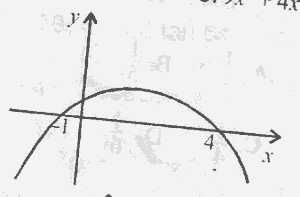From the diagram above, find the required roots/region of x.

.
A.

x$$\leq$$-1, x $$\geq$$4

B.

x$$\leq$$4

C.

-1$$\leq$$x$$\leq$$4

D.

x$$\leq$$-1, o$$\leq$$x$$\leq$$4

Explanation.
The required roots/region of x is -1$$\leq$$x$$\leq$$4